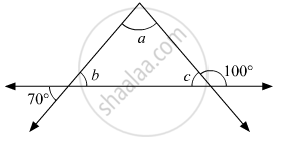Advertisement Remove all ads

# Using the Information Shown in Figure, Find the Measures of ∠ A, ∠ B and ∠ C. - Geometry

Sum

Using the information shown in figure, find the measures of ∠ a, ∠ b and ∠ c.Advertisement Remove all ads

#### Solution

c + 100 = 180                (Linear angle property)
⇒ c = 80
Now, b = 70∘     (Vertically opposite angles)
a + b + c = 180       (Angle sum property)
⇒ a + 70∘ + 80∘ = 180
⇒ a + 150∘ = 180
⇒ a = 30
Hence, the values of a, b and c are 30, 70 and 80respectively.

Is there an error in this question or solution?
Advertisement Remove all ads

#### APPEARS IN

Balbharati Mathematics 2 Geometry 9th Standard Maharashtra State Board
Chapter 3 Triangles
Practice Set 3.1 | Q 9 | Page 28
Advertisement Remove all ads
Advertisement Remove all ads
Share
Notifications

View all notifications

Forgot password?